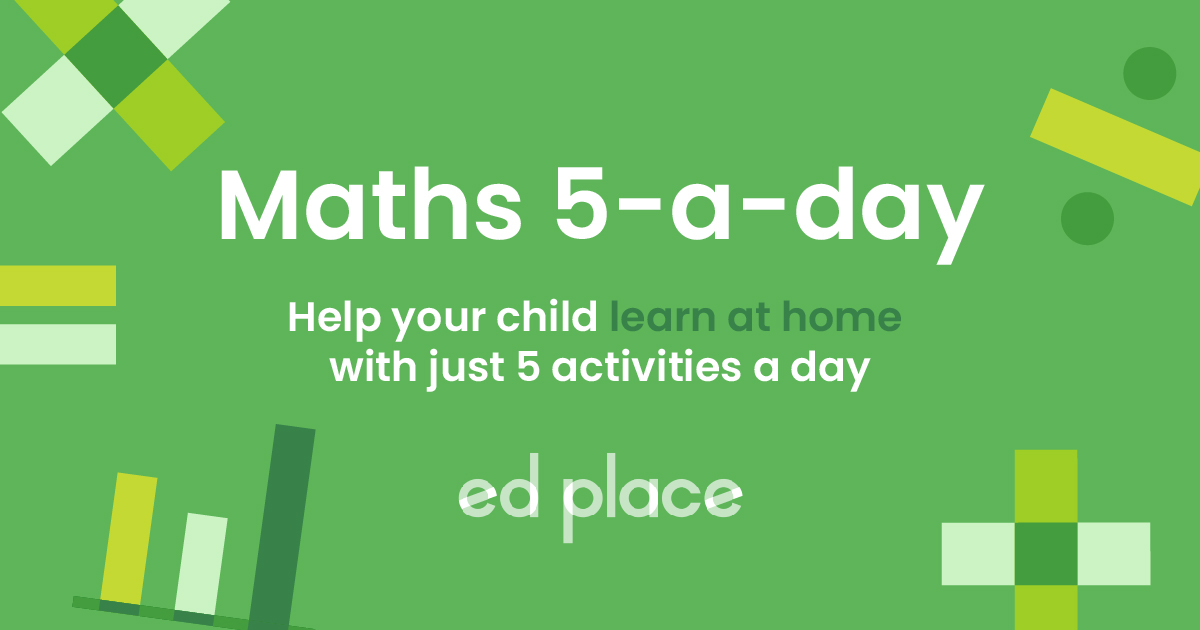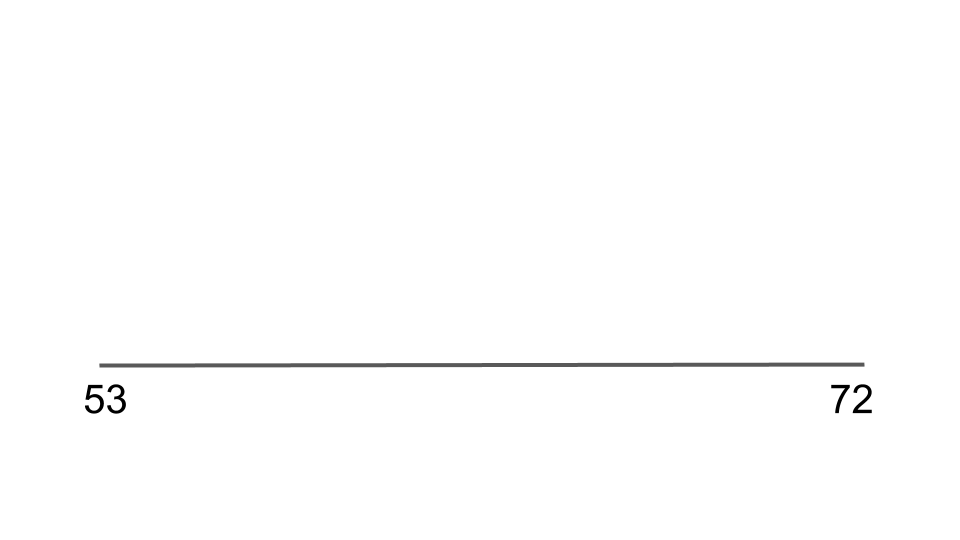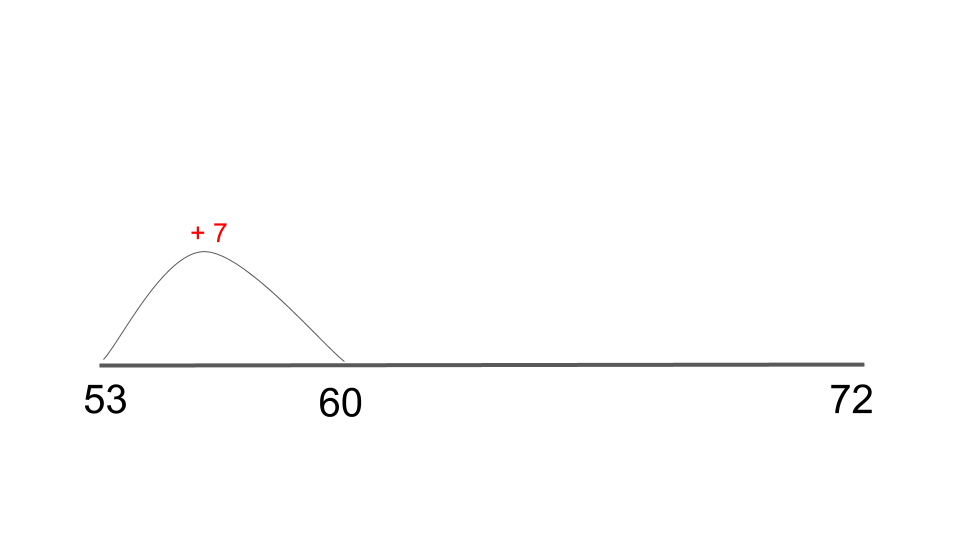# EdPlace's Year 2 Home Learning Maths Lesson: Finding the difference (subtraction) using a number line

Looking for short lessons to keep your child engaged and learning? Our experienced team of teachers have created English, maths and science lessons for the home, so your child can learn no matter where they are.  And, as all activities are self-marked, you really can encourage your child to be an independent learner. Get them started on the lesson below and then jump into our teacher-created activities to practice what they've learnt. We've recommended five to ensure they feel secure in their knowledge - 5-a-day helps keeps the learning loss at bay (or so we think!).

Are they keen to start practising straight away? Head to the bottom of the page to find the activities.

Now...onto the lesson!

Key Stage 1 Statutory Requirements for Maths
Year 2 students should be able to add and subtract numbers using concrete objects, pictorial representations, and mentally, including: two-digit numbers

# Does your child find counting backwards difficult?

Did you know that you can work out all subtraction questions using addition?

When we were in school maths was taught very differently. You are probably left wondering why they seem to have made all the methods so much more complicated!! The reason behind that is before the children are taught the quick methods they are taught methods will which help to develop their understanding of number which in turn can help develop their mental abilities.

We are here to help break down some of these methods that appear complicated so that you can understand them. Once you understand them you will be able to help your child understand them. (Or maybe you just want to understand what your child is talking about!)

1) Understand the objective of finding the difference (subtraction) on a number line.

2) Apply this knowledge to answer a variety of questions.

3) Explain to you how they completed a finding the difference question!

## Step 1 - The vocabulary!

Just like when we teach the children, we are going to go through the meaning of the terminology that will be used to teach this topic.

Subtraction - taking numbers away.

Finding the difference - (a way of working out subtraction) taking the smallest number and counting up to the biggest number to find the difference between the two numbers.

Number bonds to 10 - these are the number bonds to 10 that your child should be familiar with:

0 + 10 = 10

1 + 9 = 10

2 + 8 = 10

3 + 7 = 10

4 + 6 = 10

5 + 5 = 10

6 + 4 = 10

7 + 3 = 10

8 + 2 = 10

9 + 1 = 10

10 + 0 = 10

## Step 2 - Example 1

One of the skills we need to be able to work out the difference is to use our number bonds to be able to jump to the next multiple of 10.

The multiples of 10 are all the numbers in the 10 times table; 10, 20, 30, 40, 50, 60, 70, 80, 90, 100, 110 etc. When we talk about jumping to the next multiple of ten, these are the numbers we are referring to.

Say we were looking at the number 54, what is the multiple of 10 after 54? It is 60. We can then use our number bonds to work out how many numbers we need to add on to get to 60. We just look at the 4. We know that 4 + 6 = 10 so that we need to add 6 to get to the next multiple of 10.

## Step 3 - Use the knowledge above to help us answer subtraction questions on a number line

Let’s look at 72 - 53 = as our example.

First, we draw our line and we always start on the smallest number and end on the biggest. So we write them as below:Now we need to find the difference and we do this by jumping along our number line. We have started on 53, what is the multiple of 10 after 53? It is 60, so we write that number next on our line. Now to jump from 53 to 60, using our numbers bonds we know that 3 + 7 = 10 so adding 7 will get us to 60. This will be written like this:Now we are on 60 we can easily jump up to the next multiple of 10 by adding 10. This next jump will get us to 70. Then we have one jump left, to get from 70 to 72, which is adding 2. So this will be written as below:This means we only need to be able to do quite simple calculations to get our answer. Now all that is left to do is to find the difference. How many jumps did we do to get from 53 to 72? We now add the 7, 10 and 2 which makes 19.

So 72 - 53 = 19

Methods like this really help a child to understand the numbers they are working with and can enable them to use these methods to work out answers mentally.

## Step 4 - Putting it into practise...

The best way to learn a new skill is to practise it! Using the above knowledge have a go at answering these questions:

1. 40 - 25 =

2. 32 - 16 =

3. 74 - 43 =

4. Sophie had 64 crayons. She lost 36 of them! How many crayons does she have left?

## Step 5 - Give it a go...

Now that you’ve covered this lesson together, why not put this to the test and assign your child the following multiplication activities in this order? All activities are created by teachers and automatically marked. Plus, with an EdPlace subscription, we can automatically progress your child at a level that's right for them. Sending you progress reports along the way so you can track and measure progress, together - brilliant!

1. 15

2. 16

3. 31

4. 28 crayons

Keep going! Looking for more activities, different subjects or year groups?

Click the button below to view the EdPlace English, maths, science and 11+ activity library